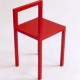[SOLVED] Is composition of measurable functions measurable?

We know that if $f: E \to \mathbb{R}$ is a Lebesgue-measurable function and $g: \mathbb{R} \to \mathbb{R}$ is a continuous function, then $g \circ f$ is Lebesgue-measurable. Can one replace the continuous function $g$ by a Lebesgue-measurable function without affecting the validity of the previous result?@Alp Uzman 2016-10-15 21:35:41

As an addition to the other answers, I think it is worth noting that in terms of imitating the relation between a topology and a continuous function when thinking of the relation between a $\sigma$-algebra and a measurable function, we can only go so far as having $g$ Borel measurable.

Let $\mathcal{T}$ be the open sets, $\mathcal{B}$ be the Borel sets and $\mathcal{M}$ be the Lebesgue measurable sets of the real line, respectively. Then we have that $\mathcal{T}\subset\mathcal{B}\subset\mathcal{M}$.

We call $f:E\to\mathbb{R}$ continuous if $f^{-1}(\mathcal{T})\subseteq\mathcal{T}$, Borel measurable if $f^{-1}(\mathcal{T})\subseteq\mathcal{B}$ and Lebesgue measurable if $f^{-1}(\mathcal{T})\subseteq\mathcal{M}$, respectively. Accompanying the inclusions of the classes of sets, we have that every continuous function is Borel measurable and every Borel measurable function is Lebesgue measurable.

It turns out that $f$ is Borel iff $f^{-1}(\mathcal{B})\subseteq\mathcal{B}$, which is analogous to the continuous case, but we don't have $f^{-1}(\mathcal{M})\subseteq\mathcal{M}$ in general for Lebesgue measurable functions $f$. The best we can do is that $f$ is Lebesgue measurable iff $f^{-1}(\mathcal{B})\subseteq\mathcal{M}$.

Consequently if $f$ is Lebesgue measurable and $g$ is Borel measurable, then $g\circ f$ is Lebesgue measurable (just like in the continuous case), and if $f,g$ are both Borel measurable then $g\circ f$ is Borel measurable (again, just like in the continuous case), but if both $f,g$ are Lebesgue measurable we can cook up examples where $g\circ g$ is not Lebesgue measurable. (For the sake of completeness, if $g$ is Lebesgue and $f$ is Borel then $g\circ f$ need not be Lebesgue: in the standard example above f is a homeomorphism).@Marine Galantin 2019-03-13 16:08:32

Hi! Thank you for the explanation, it is a great complement in order to get the thing. Please, can you tell me why the case M to M is not working for lebesgue measurable? Is this a too light restriction for such functions? Are there too many such functions?@Alp Uzman 2019-03-17 19:25:28

To the contrary, it's too strict, even at the syntactic level: indeed, since $\mathcal{B}\subsetneq\mathcal{M}$, $\{f|f^{-1}\mathcal{M}\subseteq\mathcal{M}\}\subsetneq\{f|f^{-1}\mathcal{B}\subseteq\mathcal{M}\}$.@Alp Uzman 2019-03-17 19:27:23

Here is one way to think about this: By the standard example above presented by Mirjam we know that there are homeomorphisms of closed intervals that fail to take a leb-measurable set to another leb-measurable set under preimages. Thus if we defined Lebesgue measurability of $f$ as the requirement that $f^{-1}\mathcal{M}\subseteq \mathcal{M}$, we would have to be content with continuous functions not being measurable; which in the standard way of developing integrals would prohibit one to integrate a continuous function; which in turn means that we would have to let calculus go.@Alp Uzman 2019-03-17 19:28:49

(There is nothing wrong with letting calculus go per se, though unless you have a better idea the number of your followers will be very limited.)@Mirjam 2013-01-21 16:27:54

Here is the standard example:

Let $f\colon [0,1]\to [0,1]$ be the Cantor–Lebesgue function. This is a monotonic and continuous function, and the image $f(C)$ of the Cantor set $C$ is all of $[0,1]$. Define $g(x) = x + f(x)$. Then $g\colon [0,1] \to [0,2]$ is a strictly monotonic and continuous map, so its inverse $h = g^{-1}$ is continuous, too.

Observe that $g(C)$ measure one in $[0,2]$: this is because $f$ is constant on every interval in the complement of $C$, so $g$ maps such an interval to an interval of the same length. It follows that there is a non-Lebesgue measurable subset $A$ of $g(C)$ (Vitali's theorem: a subset of $\mathbb{R}$ is a Lebesgue null set if and only if all its subsets are Lebesgue measurable).

Put $B = g^{-1}(A) \subset C$. Then $B$ is a Lebesgue measurable set as a subset of the Lebesgue null set $C$, so the characteristic function $1_B$ of $B$ is Lebesgue measurable.

The function $k = 1_B \circ h$ is the composition of the Lebesgue measurable function $1_B$ and and the continuous function $h$, but $k$ is not Lebesgue measurable, since $k^{-1}(1) = (1_B \circ h)^{-1}(1) = h^{-1}(B) = g(B) = A$.@man_in_green_shirt 2016-08-17 15:40:22

Should the last line read $k^{-1}(B) = (1_B \circ h)^{-1}(B) = h^{-1}(B) = g(B) = A$@John Cataldo 2018-03-21 17:30:43

@man_in_green_shirt no because $1_B^{-1}(1)=B$This Wikipedia article may be what you are looking for.

According to the article, a function $f: \mathbb{R} \to \mathbb{R}$ is said to be Lebesgue-measurable if and only if for every Borel-measurable subset $B$ of $\mathbb{R}$, its pre-image ${f^{\leftarrow}}[B]$ is a Lebesgue-measurable subset of $\mathbb{R}$. Therefore, because we are dealing with two different $\sigma$-algebras of $\mathbb{R}$ here, the composition of two Lebesgue-measurable functions is not necessarily Lebesgue-measurable. This is precisely what GEdgar and AD. have mentioned.@Mohamed 2013-01-21 15:22:32

This details the Ilya comment:

Yes. In general let $(E_1,\mathcal T_l) ,(E_2,\mathcal T_2),(E_3,\mathcal T_3)$ be three meurable spaces and $f:E_1 \to E_2$ and $g:E_2 \to E_3$ mesurables functions.If $X \in \mathcal T_3$, then , by mesurability of $g$ we have : $g^{-1}(X) \in \mathcal T_2$ and by mesurability of $f$ we have $f^{-1}(g^{-1}(X)) \in \mathcal T_1$. Since $(g \circ f)^{-1} (X)=f^{-1} ( g^{-1}(X))$ we have :$$\forall X\in \mathcal T_3 \quad (g \circ f)^{-1} (X) \in \mathcal T_1$$
This gives mesurability of $g \circ f$@GEdgar 2013-01-21 15:46:34

As A.D. notes, this is probably not what the OP means. Since he now mentions "Lebesgue measurable function".

[SOLVED] Composition of 2 Lebesgue measurable functions is not lebesgue measurable: Are these two functions Borel Measurable?

• 2015-06-14 01:03:16
• illysial
• 620 View
• 2 Score
• Tags:   measure-theory

[SOLVED] Composition of measurable function and continuous function

• 2012-11-12 15:28:32
• mshj
• 2545 View
• 8 Score
• Tags:   measure-theory